# An introduction to Bayes’ theorem

## A simple explanation of Bayes’ probability theorem for data science learners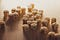Photo by Riho Kroll on Unsplash

real life, we can know the number of occurrences of an event relative to other events. For example, let's throw a fair dice. We understand that each face has an equal chance to appear, so we say that the theoretical probability of getting any face is the inverse of the number of faces, i.e., 1/6 as the regular dice has six faces.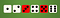Image by Author

If we colored the faces that show an odd prime number in red (i.e., 3,5), we have two red faces and four white faces. If we throw the dice, the probability of having a red face will be two faces /6 total faces, 2/6=1/3. Similarly, the probability of getting a white face when rolling the dice is 4/6 or 2/3.

If someone rolled the colored dice and got a red face, what probability will it be divisible by three? To answer this, we should divide it into two sequential problems, the first to get the probability of being red (1/3 from the previous paragraph), and the probability of being red AND divisible by three; in other words: the probability of being divisible by three GIVEN the face is red. The red faces are only two faces {3, 5}, so the only face divisible by three is {3}, which is one of two face (half the faces, or 1/2). So, the probability of having the face red AND divisible by three IS the probability of getting the number 3, which is one face out of six faces or 1/6. In other words, we can obtain the same result by multiplying the probability of being red BY the probability of being divisible by three GIVEN the face is red; i.e., 1/3 * 1/2 = 1/6.

We can write the answer in the form of equations as follows: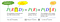Image by Author

Notice the names of the joint probability, the marginal probability, and the conditional probability. Additionally, we can define the operator “~,” which read “NOT,” so the Red faces are NOT White (~W), so we can write the previous equation as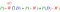Image by Author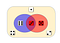Image by Author

What if we the question differently: What is the probability of getting a divisible number by three AND a red face? The numbers that are divisible by three are {3, 6}; their probability is 2/6=1/3, while the red-face numbers are {3, 5}; the probability of being red face GIVEN divisible by three is {3}/{3, 6}=1/2. Thus, P(D∩R)=P(D)×P(R|D)=1/3×1/2=1/6

We notice that it has the same result, i.e., P(R∩D)=P(D∩R), which is noticeable from the figure above.

Looks good! Right?

What did you notice? Nothing? No problem. But Thomas Bayes Noticed something good!

We can rearrange the two equations to find something interesting.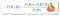Bayes theorem of conditional probability (Image by Author)

Let’s use this formula to solve another simple problem: If we throw the colored dice, what is the probability of the number to be even(E), given the face color is white(W)? If the probability of the white face given even number is 100%?

We will use the Bayes theorem to solve the problem.

You can read this as the probability of Even given White = the marginal probability of Even × the conditional probability of Whiteface given Even number ÷ White's marginal probability.

We can obtain the same result by building some useful tables, one for the events’ count and one for the probabilities: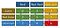The count of each marginal event (blue or yellow) and joint events (green). [Image by Author]

Notice that the sum of each row and column are written at the edges of the table. To convert this table to a table of probabilities, we should divide each number by the total number of occurrences, which is 6.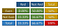Image by Author

Notice that the red cell should always be 100%. This table, however, is sometimes called the confusion matrix or the conjoint table. To generalize, we can write the probability name of each box as follows: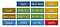Image by Author

This table shows the marginal probabilities and the joint probabilities, but what about the conditional probabilities. It is embedded inside the green boxes as follows: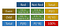Image by Author

Now I think it is clearer. Let’s play with a harder problem.

If we have the people of a city who have 1% allergy of something. We got a test kit that gives 85% true-positives and 7% false-positives. So, what is the probability for the test to give the result ‘positive’, and what is the probability for that to be true?

True-positive means that the test kit gives a positive result for those who are really allergic, while false-positive means that the test yields positive results for those who are not allergic. Similarly, you can define the true-negative and false-negative results.

Now we start by building the probability table: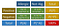Image by Author

What do we have here? What are the givens?

We have the marginal probability of the allergy, P(A), which is 1%, then we have the probability of not allergic as well, P(~A), which is 100-1= 99%. The test-kit gives 85% true-positives, which is the probability of positive, given allergic, or P(P|A)=85%. Also, we have 7% false-positives, which means the probability of positive results when the patient is NOT allergic; i.e., P(P|~A)=7%. Let us put these results in the table.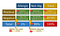The sequence of filling the probability table [Image by Author]

As we see, we start with filling the table as the sequence shows, Starting with P(A)=1%, then P(~A)=99%, then in box#3, P(A)=1%, and P(P|A)=85%, then we calculate P(A∩B) as their product = 0.85%, similarly in box#4, then we calculate the sum of 3 and 4 in box#5. The Negative row is calculated by subtracting the positive row from the total row, as in #6 and #7. Finally, calculate box #8 by either subtracting rows or by summing columns: 100%-7.78%= 0.15%+92.07%=92.22%.

From this table, we can solve the problem easily. The test's probability of giving the result (positive) =P(P)=7.78%, where 0.85% are true, and 6.93% are false. However, if we judge the positive results only, we can say that 0.85/7.78 = 10.92% of the positive results reflect real positives! Or, by Bayes rule: P(A|P)=[P(A)*P(P|A)]/[P(P)] = [1%*85%]/[7.78%]= 0.85/7.78= 10.92%. As you saw, we calculated the probability of allergic given positive from the probability of positive given allergic, which is the core of this rule.

# For Training:

1. In the park, we often see smoke (30%), mostly from normal activities like barbecues. Rarely, dangerous fires happen (0.5%); however, 8% of these fires are without smoke! If we see a cloud of smoke, what is the probability to be of a dangerous fire?
2. We put 100 balls in a box. 40 of them were plastic, and the others were glass. The balls were either white or red; 12 of the plastic balls were red, and there were 70 white balls in total. Two Questions: a. If we draw a ball randomly, it was made of glass, what is the probability of it to be of white color? And b. If we randomly draw a ball, it was red; what is its probability, not plastic?

# Summary

We showed what the Bayes theorem is and how to calculate marginal, joint, and conditional probabilities.

## About the author:

All comments, corrections, and suggestions are most welcomed

# References and further reading

## Analytics Vidhya

Analytics Vidhya is a community of Analytics and Data…

Thanks to Elliot Gunn

## Sign up for Analytics Vidhya News Bytes

### By Analytics Vidhya

Latest news from Analytics Vidhya on our Hackathons and some of our best articles! Take a look.

By signing up, you will create a Medium account if you don’t already have one. Review our Privacy Policy for more information about our privacy practices.

Check your inbox
Medium sent you an email at to complete your subscription.

Written by

## Dr Mohammad El-Nesr

Researcher and Data Analyst

## Analytics Vidhya

Analytics Vidhya is a community of Analytics and Data Science professionals. We are building the next-gen data science ecosystem https://www.analyticsvidhya.com

Written by

## Dr Mohammad El-Nesr

Researcher and Data Analyst## Analytics Vidhya

Analytics Vidhya is a community of Analytics and Data Science professionals. We are building the next-gen data science ecosystem https://www.analyticsvidhya.com

## More From Medium

Medium is an open platform where 170 million readers come to find insightful and dynamic thinking. Here, expert and undiscovered voices alike dive into the heart of any topic and bring new ideas to the surface. Learn more

Follow the writers, publications, and topics that matter to you, and you’ll see them on your homepage and in your inbox. Explore

If you have a story to tell, knowledge to share, or a perspective to offer — welcome home. It’s easy and free to post your thinking on any topic. Write on Medium